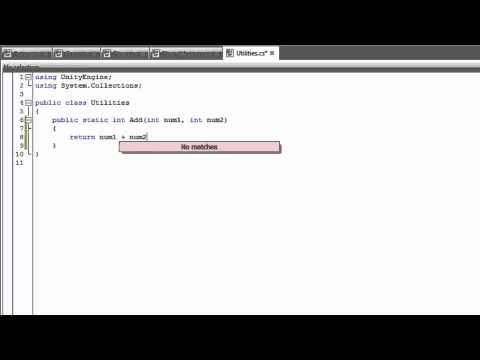# Statics

#### Difficulté: Intermédiaire

How to create static variables, methods, and classes.## Statics

Intermédiaire Scripting

### Code snippet

``````using UnityEngine;
using System.Collections;

public class Enemy
{
//Static variables are shared across all instances
//of a class.
public static int enemyCount = 0;

public Enemy()
{
//Increment the static variable to know how many
//objects of this class have been created.
enemyCount++;
}
}``````
``````#pragma strict

public class Enemy
{
//Static variables are shared across all instances
//of a class.
public static var enemyCount : int = 0;

function Enemy()
{
//Increment the static variable to know how many
//objects of this class have been created.
enemyCount++;
}
}``````
``````import UnityEngine
import System.Collections

public class Enemy:

//Static variables are shared across all instances
//of a class.
public static enemyCount = 0

public def constructor():
//Increment the static variable to know how many
//objects of this class have been created.
enemyCount += 1``````

### Code snippet

``````using UnityEngine;
using System.Collections;

public class Game
{
void Start ()
{
Enemy enemy1 = new Enemy();
Enemy enemy2 = new Enemy();
Enemy enemy3 = new Enemy();

//You can access a static variable by using the class name
//and the dot operator.
int x = Enemy.enemyCount;
}
}``````
``````#pragma strict

public class Game
{
function Start ()
{
var enemy1 = new Enemy();
var enemy2 = new Enemy();
var enemy3 = new Enemy();

//You can access a static variable by using the class name
//and the dot operator.
var x : int = Enemy.enemyCount;
}
}``````
``````import UnityEngine
import System.Collections

public class Game:

private def Start():
enemy1 = Enemy()
enemy2 = Enemy()
enemy3 = Enemy()

//You can access a static variable by using the class name
//and the dot operator.
x as int = Enemy.enemyCount``````

### Code snippet

``````using UnityEngine;
using System.Collections;

public class Player : MonoBehaviour
{
//Static variables are shared across all instances
//of a class.
public static int playerCount = 0;

void Start()
{
//Increment the static variable to know how many
//objects of this class have been created.
playerCount++;
}
}``````
``````#pragma strict

public class Player extends MonoBehaviour
{
//Static variables are shared across all instances
//of a class.
public static var playerCount : int = 0;

function Start()
{
//Increment the static variable to know how many
//objects of this class have been created.
playerCount++;
}
}``````
``````import UnityEngine
import System.Collections

public class Player(MonoBehaviour):

//Static variables are shared across all instances
//of a class.
public static playerCount = 0

private def Start():
//Increment the static variable to know how many
//objects of this class have been created.
playerCount += 1``````

### Code snippet

``````using UnityEngine;
using System.Collections;

public class PlayerManager : MonoBehaviour
{
void Start()
{
//You can access a static variable by using the class name
//and the dot operator.
int x = Player.playerCount;
}
}``````
``````#pragma strict

function Start ()
{
//You can access a static variable by using the class name
//and the dot operator.
var x : int = Player.playerCount;
}``````
``````import UnityEngine
import System.Collections

public class PlayerManager(MonoBehaviour):

private def Start():
//You can access a static variable by using the class name
//and the dot operator.
x as int = Player.playerCount``````

### Code snippet

``````using UnityEngine;
using System.Collections;

public static class Utilities
{
//A static method can be invoked without an object
//of a class. Note that static methods cannot access
//non-static member variables.
public static int Add(int num1, int num2)
{
return num1 + num2;
}
}``````
``````#pragma strict

public static class Utilities
{
//A static method can be invoked without an object
//of a class. Note that static methods cannot access
//non-static member variables
public static function Add(num1 : int, num2 : int) : int
{
return num1 + num2;
}
}``````
``````import UnityEngine
import System.Collections

public static class Utilities:

//A static method can be invoked without an object
//of a class. Note that static methods cannot access
//non-static member variables.
public static def Add(num1 as int, num2 as int) as int:
return (num1 + num2)``````

### Code snippet

``````using UnityEngine;
using System.Collections;

public class UtilitiesExample : MonoBehaviour
{
void Start()
{
//You can access a static method by using the class name
//and the dot operator.
int x = Utilities.Add (5, 6);
}
}``````
``````#pragma strict

function Start ()
{
//You can access a static method by using the class name
//and the dot operator.
var x : int = Utilities.Add (5, 6);
}``````
``````import UnityEngine
import System.Collections

public class UtilitiesExample(MonoBehaviour):

private def Start():
//You can access a static method by using the class name
//and the dot operator.
x as int = Utilities.Add(5, 6)``````